# Directions: Preview (skim through) the packet to help you complete the following. Fill in the title of each topic in the boxes provided

 Page 5/5 Date 23.04.2018 Size 0.59 Mb. #45993
1   2   3   4   5
Endothermic Example: H2 (g) + I2 (g) + heat  2 HI (g)

Low PE + heat  High PE

Total E before = Total E after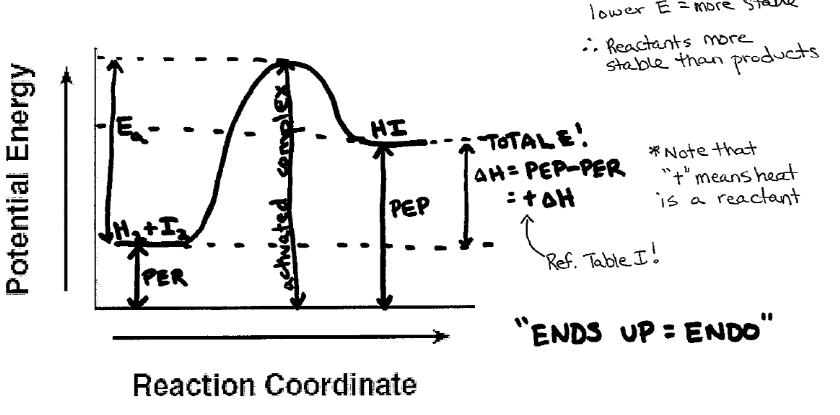ENTROPY NOTES:
Entropy is a measure of disorder in a system. Nature prefers disorder. Solids are more ordered than liquids and gases. If more gas exists when the change is complete then there is more disorder in the system, hence an increase in entropy. A solution (aq) is more disordered than a solid or liquid.
Entropy can be increased by:
1) increasing_ temperature (KE)

(increasing/decreasing)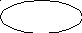ex. Which has the greatest entropy? H2O (l) @ 20°C OR H2O (l) @ 70°C

2) _ increasing _ the number of particles (increasing/decreasing)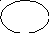ex. Which has the greatest entropy? 5 H2 (g) OR 2 H2 (g)

3) phase changes S  L  Aq  G

entropy increasesex. Which has the greatest entropy? CO2 (s) ↔ CO2 (g)

Entropy Practice: Circle the side that has the GREATEST entropy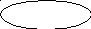1. 4 Al (s) + 3 O2 (g)  2 Al2O3 (s)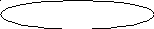1. Cu (s) + 2 HNO3 (aq)  CuNO3 (aq) + NO2 (g) + H2O (l)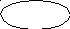1. N2 (g) + 3 H2 (g)  2 NH3 (g)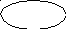1. NaCl at 50oC  NaCl at 30oC

REVIEW:

Matter is not created or destroyed, it just changes form. What this means to chemists is that if 126 carbon atoms are put into a reaction, we had better get 126 carbon atoms out when the reaction is over. The carbon atoms may be bonded to hydrogen atoms when the reaction started and bonded to oxygen atoms when the reaction was over. This doesn’t matter, what matters is that all of the atoms are accounted for.

Anatomy of a chemical reaction:

• Every chemical reaction has one or more reactants and one or more products.

• The reactants are always written on the left and the products are written on the right.

• The reactants and products are separated by and arrow.

DEMO/EXAMPLE:

The following is a reaction that occurs relatively quickly when aluminum foil is added to

copper (II) chloride solution: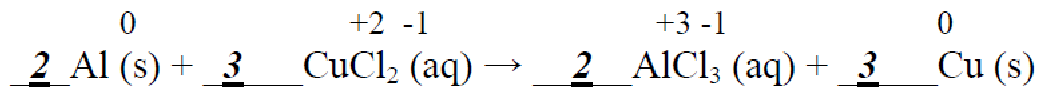1.) Balance the equation.

2.) Assign charges to each of the elements and ions by writing them above each in the equation.

3.) Explain how this equation demonstrates conservation of mass and charge.

There are the same number of each type of atom in the reactants and products, which demonstrates conservation of mass. The aluminum atoms each lose 3 electrons, for a total of 6 electrons lost, and the copper atoms each gain 2 electrons, for a total of 6 electrons gained; this demonstrates conservation of charge.
4.) A question on a test says, “Explain how this reaction shows the conservation of mass”. Which of the following responses is best and why?

a) There are the same number of atoms in the reactants and products.

b) The equation is balanced to show conservation of mass/matter.

c) There are the same number of aluminum and copper atoms in both the reactants and products.

d) There are the same number and type of each atom in the reactants and products.
The best response is: __d___ (abcd) because: It is an explanation (unlike choice b) that gives specific information regarding each element (unlike choices a & c).

Conservation of Energy NOTES

What about ENERGY when bonds are formed?

• Bonds allow atoms to go from unstable ( high energy) to stable ( low energy). (low / high) (low / high)

• Therefore….energy must be released ! This is an example (absorbed / released)

exothermic reaction.

(endothermic / exothermic)

• Put “energy” in its proper place (either reactant or product) in the reaction below: 2 H2 + O2 2 H2O + heat

What about ENERGY when bonds are broken?

• Bonds allow atoms to go from stable ( low energy) to unstable ( high energy). (low / high) (low / high)

• Therefore….energy must be absorbed ! This is an example (absorbed / released)

endothermic reaction.

(endothermic / exothermic)

• Put “energy” in its proper place (either reactant or product) in the reaction below: 2 H2O + heat 2 H2 + O2 Practice Questions:

1.) Which quantities must be conserved in all chemical reactions?

(1) mass, charge, density (2) charge, volume, density (3) mass, charge, energy (4) charge, volume, energy

2.) During all chemical reactions, mass and energy are

(1) absorbed (2) conserved (3) formed (4) released

3.) Which equation represents an exothermic reaction?

(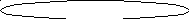1) H2O(s) + 6.01 kJ → H2O(l) (3) H2(g) + I2(g) + 53.0 kJ → 2HI(g)

(2) 2H2(g) + O2(g) → 2H2O(g) + 483.6 kJ (4) N2(g) + 2O2(g) + 66.4 kJ → 2NO2(g)
4.) Given the balanced equation representing a reaction:

H2(g) + Cl2(g) →2HCl(g) + energy

Which statement describes the energy changes in this reaction?

(1) Energy is absorbed as bonds are formed, only.

(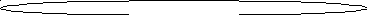2) Energy is released as bonds are broken only.

(3) Energy is absorbed as bonds are broken, and energy is released as bonds are formed.

(4) Energy is absorbed as bonds are formed, and energy is released as bonds are broken.
5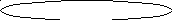.) Which statement describes what occurs as two atoms of bromine combine to become a molecule of bromine?

(1) Energy is absorbed as a bond is formed. (3) Energy is released as a bond is formed.

(2) Energy is absorbed as a bond is broken. (4) Energy is released as a bond is broken.

Bonding Reactions & Particle Drawings Practice
Directions: Using the given word statement, determine the chemical equation. You will then draw a particle diagram, balance it, and then create a balanced chemical equation.
Bonding Situation #1: hydrogen molecules react with nitrogen molecules to form ammonia (NH3)
Basic/Unbalanced Equation: H2(g) + N2 (g) NH3

Draw your reactants in the “Reactants” box and your products in the “Products” box.

Then, balance the particles:

 Key: =N = H

Reactants Box Products Box

(“BEFORE” reaction) (“AFTER” reaction)

_3__ molecules of H2 + _1__ molecules of N2 formed into _2__ molecules of NH3
Balanced Equation: 3 H2 + N2 2 NH3

Bonding Situation #2: Lithium atoms react with chlorine molecules to form lithium chloride

Basic/Unbalanced Equation: Li + Cl2 LiCl

 Key: =Li = Cl

Reactants Box Products Box

(“BEFORE” reaction) (“AFTER” reaction)

_2__ atoms of Li + _1__ molecules of Cl2 formed into _2__ units of LiCl
Balanced Equation: 2 Li + Cl2 2 LiCl

Directory: site -> handlers